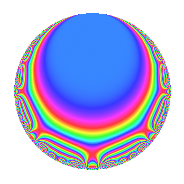# Properties

 Label 4020.2.a.bLevel 4020 Weight 2 Character orbit 4020.a Self dual Yes Analytic conductor 32.100 Analytic rank 1 Dimension 4 CM No Inner twists 1

# Learn more about

## Newspace parameters

 Level: $$N$$ = $$4020 = 2^{2} \cdot 3 \cdot 5 \cdot 67$$ Weight: $$k$$ = $$2$$ Character orbit: $$[\chi]$$ = 4020.a (trivial)

## Newform invariants

 Self dual: Yes Analytic conductor: $$32.0998616126$$ Analytic rank: $$1$$ Dimension: $$4$$ Coefficient field: 4.4.9301.1 Coefficient ring: $$\Z[a_1, \ldots, a_{7}]$$ Coefficient ring index: $$1$$ Fricke sign: $$1$$ Sato-Tate group: $\mathrm{SU}(2)$

## $q$-expansion

Coefficients of the $$q$$-expansion are expressed in terms of a basis $$1,\beta_1,\beta_2,\beta_3$$ for the coefficient ring described below. We also show the integral $$q$$-expansion of the trace form.

 $$f(q)$$ $$=$$ $$q$$ $$- q^{3}$$ $$- q^{5}$$ $$+ ( \beta_{1} + \beta_{2} ) q^{7}$$ $$+ q^{9}$$ $$+O(q^{10})$$ $$q$$ $$- q^{3}$$ $$- q^{5}$$ $$+ ( \beta_{1} + \beta_{2} ) q^{7}$$ $$+ q^{9}$$ $$+ ( 1 + \beta_{1} ) q^{11}$$ $$+ ( -1 - \beta_{1} - \beta_{2} - \beta_{3} ) q^{13}$$ $$+ q^{15}$$ $$+ ( \beta_{2} + \beta_{3} ) q^{17}$$ $$+ ( -1 - \beta_{1} + \beta_{3} ) q^{19}$$ $$+ ( -\beta_{1} - \beta_{2} ) q^{21}$$ $$+ ( 1 - 2 \beta_{1} - 2 \beta_{2} ) q^{23}$$ $$+ q^{25}$$ $$- q^{27}$$ $$+ ( -1 + \beta_{1} + \beta_{2} - 2 \beta_{3} ) q^{29}$$ $$+ ( -4 - \beta_{1} - 2 \beta_{2} - \beta_{3} ) q^{31}$$ $$+ ( -1 - \beta_{1} ) q^{33}$$ $$+ ( -\beta_{1} - \beta_{2} ) q^{35}$$ $$+ ( 1 + 2 \beta_{3} ) q^{37}$$ $$+ ( 1 + \beta_{1} + \beta_{2} + \beta_{3} ) q^{39}$$ $$+ ( -2 - 3 \beta_{1} - 2 \beta_{2} + 3 \beta_{3} ) q^{41}$$ $$+ ( 4 - \beta_{1} - \beta_{2} + \beta_{3} ) q^{43}$$ $$- q^{45}$$ $$+ ( 1 - \beta_{1} + \beta_{2} - 3 \beta_{3} ) q^{47}$$ $$+ ( -1 + 3 \beta_{1} + \beta_{3} ) q^{49}$$ $$+ ( -\beta_{2} - \beta_{3} ) q^{51}$$ $$+ ( 1 + 3 \beta_{1} - 2 \beta_{2} - \beta_{3} ) q^{53}$$ $$+ ( -1 - \beta_{1} ) q^{55}$$ $$+ ( 1 + \beta_{1} - \beta_{3} ) q^{57}$$ $$+ ( 3 - 3 \beta_{1} + 3 \beta_{2} + \beta_{3} ) q^{59}$$ $$+ ( -3 - \beta_{1} + 4 \beta_{2} - 3 \beta_{3} ) q^{61}$$ $$+ ( \beta_{1} + \beta_{2} ) q^{63}$$ $$+ ( 1 + \beta_{1} + \beta_{2} + \beta_{3} ) q^{65}$$ $$+ q^{67}$$ $$+ ( -1 + 2 \beta_{1} + 2 \beta_{2} ) q^{69}$$ $$+ ( -3 - 4 \beta_{1} + \beta_{2} - 6 \beta_{3} ) q^{71}$$ $$+ ( 4 \beta_{1} - 3 \beta_{2} + \beta_{3} ) q^{73}$$ $$- q^{75}$$ $$+ ( 3 + 3 \beta_{1} + 2 \beta_{2} + \beta_{3} ) q^{77}$$ $$+ ( 3 \beta_{1} - \beta_{2} + 3 \beta_{3} ) q^{79}$$ $$+ q^{81}$$ $$+ ( 5 - \beta_{2} + 2 \beta_{3} ) q^{83}$$ $$+ ( -\beta_{2} - \beta_{3} ) q^{85}$$ $$+ ( 1 - \beta_{1} - \beta_{2} + 2 \beta_{3} ) q^{87}$$ $$+ ( -7 + 2 \beta_{1} - \beta_{2} ) q^{89}$$ $$+ ( -6 - 5 \beta_{1} - \beta_{2} + \beta_{3} ) q^{91}$$ $$+ ( 4 + \beta_{1} + 2 \beta_{2} + \beta_{3} ) q^{93}$$ $$+ ( 1 + \beta_{1} - \beta_{3} ) q^{95}$$ $$+ ( -6 - 3 \beta_{1} - 2 \beta_{2} ) q^{97}$$ $$+ ( 1 + \beta_{1} ) q^{99}$$ $$+O(q^{100})$$ $$\operatorname{Tr}(f)(q)$$ $$=$$ $$4q$$ $$\mathstrut -\mathstrut 4q^{3}$$ $$\mathstrut -\mathstrut 4q^{5}$$ $$\mathstrut -\mathstrut q^{7}$$ $$\mathstrut +\mathstrut 4q^{9}$$ $$\mathstrut +\mathstrut O(q^{10})$$ $$4q$$ $$\mathstrut -\mathstrut 4q^{3}$$ $$\mathstrut -\mathstrut 4q^{5}$$ $$\mathstrut -\mathstrut q^{7}$$ $$\mathstrut +\mathstrut 4q^{9}$$ $$\mathstrut +\mathstrut 5q^{11}$$ $$\mathstrut -\mathstrut q^{13}$$ $$\mathstrut +\mathstrut 4q^{15}$$ $$\mathstrut -\mathstrut 4q^{17}$$ $$\mathstrut -\mathstrut 7q^{19}$$ $$\mathstrut +\mathstrut q^{21}$$ $$\mathstrut +\mathstrut 6q^{23}$$ $$\mathstrut +\mathstrut 4q^{25}$$ $$\mathstrut -\mathstrut 4q^{27}$$ $$\mathstrut -\mathstrut q^{29}$$ $$\mathstrut -\mathstrut 11q^{31}$$ $$\mathstrut -\mathstrut 5q^{33}$$ $$\mathstrut +\mathstrut q^{35}$$ $$\mathstrut +\mathstrut q^{39}$$ $$\mathstrut -\mathstrut 13q^{41}$$ $$\mathstrut +\mathstrut 15q^{43}$$ $$\mathstrut -\mathstrut 4q^{45}$$ $$\mathstrut +\mathstrut 7q^{47}$$ $$\mathstrut -\mathstrut 3q^{49}$$ $$\mathstrut +\mathstrut 4q^{51}$$ $$\mathstrut +\mathstrut 13q^{53}$$ $$\mathstrut -\mathstrut 5q^{55}$$ $$\mathstrut +\mathstrut 7q^{57}$$ $$\mathstrut +\mathstrut q^{59}$$ $$\mathstrut -\mathstrut 15q^{61}$$ $$\mathstrut -\mathstrut q^{63}$$ $$\mathstrut +\mathstrut q^{65}$$ $$\mathstrut +\mathstrut 4q^{67}$$ $$\mathstrut -\mathstrut 6q^{69}$$ $$\mathstrut -\mathstrut 6q^{71}$$ $$\mathstrut +\mathstrut 8q^{73}$$ $$\mathstrut -\mathstrut 4q^{75}$$ $$\mathstrut +\mathstrut 9q^{77}$$ $$\mathstrut -\mathstrut q^{79}$$ $$\mathstrut +\mathstrut 4q^{81}$$ $$\mathstrut +\mathstrut 18q^{83}$$ $$\mathstrut +\mathstrut 4q^{85}$$ $$\mathstrut +\mathstrut q^{87}$$ $$\mathstrut -\mathstrut 24q^{89}$$ $$\mathstrut -\mathstrut 29q^{91}$$ $$\mathstrut +\mathstrut 11q^{93}$$ $$\mathstrut +\mathstrut 7q^{95}$$ $$\mathstrut -\mathstrut 23q^{97}$$ $$\mathstrut +\mathstrut 5q^{99}$$ $$\mathstrut +\mathstrut O(q^{100})$$

Basis of coefficient ring in terms of a root $$\nu$$ of $$x^{4}\mathstrut -\mathstrut$$ $$x^{3}\mathstrut -\mathstrut$$ $$5$$ $$x^{2}\mathstrut +\mathstrut$$ $$x\mathstrut +\mathstrut$$ $$3$$:

 $$\beta_{0}$$ $$=$$ $$1$$ $$\beta_{1}$$ $$=$$ $$\nu$$ $$\beta_{2}$$ $$=$$ $$\nu^{2} - \nu - 3$$ $$\beta_{3}$$ $$=$$ $$\nu^{3} - \nu^{2} - 4 \nu$$
 $$1$$ $$=$$ $$\beta_0$$ $$\nu$$ $$=$$ $$\beta_{1}$$ $$\nu^{2}$$ $$=$$ $$\beta_{2}\mathstrut +\mathstrut$$ $$\beta_{1}\mathstrut +\mathstrut$$ $$3$$ $$\nu^{3}$$ $$=$$ $$\beta_{3}\mathstrut +\mathstrut$$ $$\beta_{2}\mathstrut +\mathstrut$$ $$5$$ $$\beta_{1}\mathstrut +\mathstrut$$ $$3$$

## Embeddings

For each embedding $$\iota_m$$ of the coefficient field, the values $$\iota_m(a_n)$$ are shown below.

For more information on an embedded modular form you can click on its label.

Label $$\iota_m(\nu)$$ $$a_{2}$$ $$a_{3}$$ $$a_{4}$$ $$a_{5}$$ $$a_{6}$$ $$a_{7}$$ $$a_{8}$$ $$a_{9}$$ $$a_{10}$$
1.1
 −0.784476 0.869986 −1.68863 2.60312
0 −1.00000 0 −1.00000 0 −2.38460 0 1.00000 0
1.2 0 −1.00000 0 −1.00000 0 −2.24312 0 1.00000 0
1.3 0 −1.00000 0 −1.00000 0 −0.148523 0 1.00000 0
1.4 0 −1.00000 0 −1.00000 0 3.77625 0 1.00000 0
 $$n$$: e.g. 2-40 or 990-1000 Significant digits: Format: Complex embeddings Normalized embeddings Satake parameters Satake angles

## Inner twists

This newform does not admit any (nontrivial) inner twists.

## Atkin-Lehner signs

$$p$$ Sign
$$2$$ $$-1$$
$$3$$ $$1$$
$$5$$ $$1$$
$$67$$ $$-1$$

## Hecke kernels

This newform can be constructed as the kernel of the linear operator $$T_{7}^{4}$$ $$\mathstrut +\mathstrut T_{7}^{3}$$ $$\mathstrut -\mathstrut 12 T_{7}^{2}$$ $$\mathstrut -\mathstrut 22 T_{7}$$ $$\mathstrut -\mathstrut 3$$ acting on $$S_{2}^{\mathrm{new}}(\Gamma_0(4020))$$.